# 反函数f-1(y)

f-1(y) = (y-3)/2

（我用 y，而不用 x，因为它们代表不同的值。）

## 还原### 例子：

f(4) = 2×4+3 = 11

f-1(11) = (11-3)/2 = 4

f-1( f(4) ) = 4

"f 反函数 的 f 的 4 等于 4"

f-1( f(x) ) = x

f( f-1(x) ) = x

### 例子：

f-1(11) = (11-3)/2 = 4

f(4) = 2×4+3 = 11

f( f-1(11) ) = 11

"f 的  f 反函数的 11 等于 11"

## 用代数来解

 函数： f(x) = 2x+3 用 "y" 代替 "f(x)"： y = 2x+3 每边减 3: y-3 = 2x 每边除 2： (y-3)/2 = x 换边： x = (y-3)/2 结果 （用 "f-1(y)" 来代替 "x"）： f-1(y) = (y-3)/2

## 把华氏转换为摄氏

 将华氏转换为摄氏： f(F) = (F - 32) × 59 反函数（将摄氏转换为华氏）是： f-1(C) = (C × 95) + 32

## 常见函数的反函数

 反函数 小心！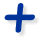<=>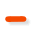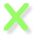<=>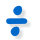不要除以零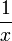<=>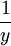x 和 y 不能是零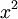<=>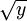x 和 y ≥ 0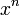<=>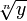or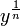n 不等于零 （n 为奇数、偶数、负数和正数都有不同的规则）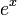<=>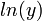y > 0<=>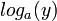y 和 a > 0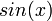<=>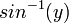-π/2 to +π/2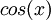<=>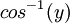0 to π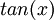<=>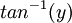-π/2 to +π/2

（注意：去阅读反正弦、余弦和正切了解更多。）

## 小心！

### 例子：平方与平方根

 平方： (-2)2 = 4 反函数（平方根）： √(4) = 2

### 例子：（续）

• x2 没有反函数
• {x2 | x ≥ 0 }（这是合建构式符号，意思是 "x 平方，满足 x 大于或等于零这个性质"）反函数。

## 没有反函数？

 一般函数 单射函数没有反函数能有反函数 如果一个 y值有多于一个 x值，还原时应该选哪个呢？ 如果每个 x值的 y值是唯一的（没有其它 x值有这个 y值），从 y 还原到 x 就只有一个选择。

"每个 x值的 y值是唯一的" 这个概念有个名字，叫 "单射"，也称 "一对一"：

## 定义域与值域简单地说，定义域是所有输入函数的值（值域的所有输出值）。

 但我们可以限制定义域，使得每个 y值只有一个唯一的 x值 ………… 这样函数就可以有反函数了： 注意： 函数 f(x) 从定义域映入到值域， 反函数 f-1(y) 从值域映入到定义域。…… 我们也可以……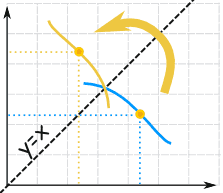f(x)f-1(x) 像彼此的镜像
（相对于对角线反转）。

f(x)f-1(x) 的图是相对于直线 y=x 对称的

### 例子：平方和平方根（续）

• {x2 | x ≥ 0 } "x 的平方，满足 x 大于或等于零的性质"
• {√x | x ≥ 0 } "x 的平方根，满足 x 大于或等于零的性质"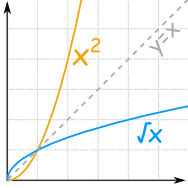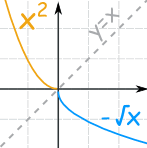• {x2 | x ≤ 0 }
• {−√x | x ≥ 0 }

y = x/2 + sin(x)

y …… ？ = x

## 记法备注

 f-1(x) …… 和 …… f(x)-1 是不同的 函数 f 的反函数 f(x)-1 = 1/f(x) （倒数）

## 总括

• f(x) 的反函数是 f-1(y)
• 我们可以把 "流程图" 倒转来求反函数
• 或者用代数来解反函数：
• 用 "y" 来代替 "f(x)"，然后
• 解 x
• 我们可能需要限制定义域才能使得函数有反函数